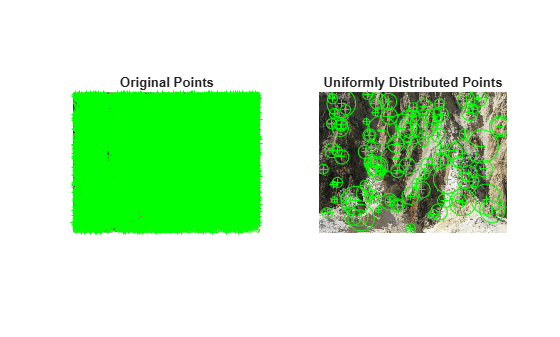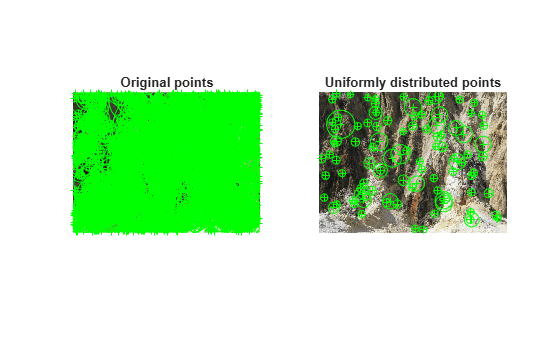selectUniform

Select uniformly distributed subset of feature points

Description

example

pointsOut = selectUniform(points,N,imageSize) returns N uniformly distributed points from pointsIn points of an image of size imageSize.

Examples

collapse all

Detect and plot a subset of uniformly distributed KAZE features from an image.

Detect and display KAZE features.

points1 = detectKAZEFeatures(im2gray(im))
points1 =
6712x1 KAZEPoints array with properties:

Location: [6712x2 single]
Metric: [6712x1 single]
Count: 6712
Scale: [6712x1 single]
Orientation: [6712x1 single]

subplot(1,2,1)
imshow(im)
hold on
plot(points1)
hold off
title('Original Points')

Select a uniformly distributed subset of points.

numPoints = 100;
points2 = selectUniform(points1,numPoints,size(im))
points2 =
100x1 KAZEPoints array with properties:

Location: [100x2 single]
Metric: [100x1 single]
Count: 100
Scale: [100x1 single]
Orientation: [100x1 single]

subplot(1,2,2)
imshow(im)
hold on
plot(points2)
hold off
title('Uniformly Distributed Points')Detect many corners by reducing the quality threshold.

points1 = detectHarrisFeatures(im2gray(im), 'MinQuality', 0.05);

Plot image with detected corners.

subplot(1,2,1);
imshow(im);
hold on
plot(points1);
hold off
title('Original points');

Select a uniformly distributed subset of points.

numPoints = 100;
points2 = selectUniform(points1,numPoints,size(im));

Plot images showing original and subset of points.

subplot(1, 2, 2);
imshow(im);
hold on
plot(points2);
hold off
title('Uniformly distributed points');Load an image into the workspace.

Detect many corners by reducing the quality threshold.

points1 = detectBRISKFeatures(im2gray(im), 'MinQuality', 0.05);

Plot image with detected corners.

subplot(1,2,1);
imshow(im);
hold on
plot(points1);
hold off
title('Original points');

Select a uniformly distributed subset of points.

numPoints = 100;
points2 = selectUniform(points1,numPoints,size(im));

Plot images showing original and subset of points.

subplot(1, 2, 2);
imshow(im);
hold on
plot(points2);
hold off
title('Uniformly distributed points');Detect and display SURF features.

points1 = detectSURFFeatures(im2gray(im));
subplot(1,2,1);
imshow(im);
hold on
plot(points1);
hold off
title('Original points');

Select a uniformly distributed subset of points.

numPoints = 100;
points2 = selectUniform(points1,numPoints,size(im));

Plot images showing original and subset of points.

subplot(1, 2, 2);
imshow(im);
hold on
plot(points2);
hold off
title('Uniformly distributed points');Input Arguments

collapse all

Points object, specified as a points object. The object contains information about the feature points detected in the input image. To obtain points, use the appropriate detect function.

Number of uniformly distributed points to select, specified as an integer.

Size of image, specified as a 2-element vector for grayscale images or a 3-element vector for truecolor images.

Introduced in R2012a